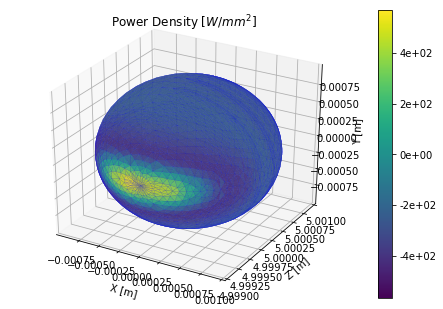# Undulator Power Density on a CAD Generated Object¶

This is a simple example of an undulator power density calculated on a CAD object. Currently OSCARS supports the STL (binary) format. This is easily exported from your typical CAD software.

This example folder contains one simple cad object file which is used below.

In :
# Plots inline for notebook
%matplotlib inline

# Import the OSCARS SR module
import oscars.sr

# Import the 3D and parametric surfaces utilities
from oscars.plots3d_mpl import *

OSCARS v2.1.8 - Open Source Code for Advanced Radiation Simulation
Brookhaven National Laboratory, Upton NY, USA
http://oscars.bnl.gov
oscars@bnl.gov

In :
# Create a new OSCARS object.  Default to 8 threads and always use the GPU if available


## Undulator Field¶

Create a simple undulator field

In :
# Clear all existing fields and create an undulator field
osr.clear_bfields()
osr.add_bfield_undulator(bfield=[0, 1, 0], period=[0, 0, 0.050], nperiods=31)


## Particle Beam and Trajectory¶

Define a particle beam, in this case a 3 [GeV] electron beam. You must also define the start and stop times for the calculation.

In :
# Define simple electron beam
osr.set_particle_beam(energy_GeV=3, x0=[0, 0, -1], current=0.5)

# Define the start and stop times for the calculation
osr.set_ctstartstop(0, 2)


## Calculate Power Density on CAD STL Surface¶

Will import the STL file and calculate the power density. The return object is different from a normal power density calculation and allows for visualization of the object.

In :
# Clculate power density on CAD surface from STL file.
# Here 'scale' is used since the input file units are in mm
# while OSCARS expects m (there is no STL standard so you must
# keep track of this yourself)
pd = osr.calculate_power_density_stl(
ifile='sphere.stl',
scale=0.001,
translation=[0, 0, 5]
)

plot_power_density_stl(pd, colorbar=True)In :
plot_power_density_stl(pd, colorbar=True)